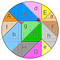# The Pizza Theorem

After a boring working day, you can’t wait to enjoy a wonderful weekend. Therefore, you invite your best friend and going to hold a party in your backyard. The table is full of delicious food and drink; everyone is excited to enjoy a good time. While you are going to cut the pizza and distribute it to the guest, some quarrel begins in the crowd, arguing the size of the pizza slice isn’t equal. Since you can’t precisely cut through the center of the circle, perhaps you can’t divide the pizza equally…

Is there any way to divide the pizza equally? Although the problem seems to be easily and lifelike, it is a famous math problem that confused and lure interest to the mathematician in the late 19 century, and finally got solved in 2012. The interesting pizza divide problem came into a famous theorem in geometry which is known as the Pizza theorem.

Here is a brief claim from the pizza theorem:

Assign an arbitrary point in the circle for the cutting center, and cut through the point for n times with an equally angular interval. Finally, we can get 2n slice of pizza, and The sum of the areas of the odd-numbered sectors equals the sum of the areas of the even-numbered sectors. ( n is an even larger than 2 , n=4,6,8,10,12…

The pizza theorem was originally proposed as a challenging problem by Upton(1967). Soon afterward, Michael Goldberg using direct manipulation of the algebraic expressions for the areas of the sectors. Furthermore, Carter & Wagon (1994) provide an alternative proof by dissection showing how to partition the sectors into smaller pieces so that each piece in an odd-numbered sector has a congruent piece in an even-numbered sector.Proof without words for 8 sectors by Carter & Wagon (1994a). ,Wiki

# Proof.

In order to confirm the theorem, we are going to calculate the area of each slice of pizza and sum the total area.

Without loss of generality, we can define a unit circle with the origin, point O, and the arbitrary cutting center, point P.

If we extend the line of OP and intersect with the circle at point Q. The first slice will go through point a0 and P, where the shift angle between the extended line and the first cut is ∠a0PQ

The length of the cross-section of slice 1,r0, will become a function of theta, depends on the angle between ai, P, Q.

Therefore, if we keep cutting the pizza through point P with an angle shift Δϕ=π/n counterclockwise, we can get slice S1, S2, S3,…S2n respectively.

Each slice of pizza’s area can be calculated by calculus easily by the following expression. However, now we are going, to sum up the odd-numbered sectors, S1, S3, S5,…S2n-1 only, to prove the Pizza theorem.

Using the summation notation makes the expression more concise.

Meanwhile, we can alter the dummy variable in the integral by changing the range of the integral.

Since the upper and down limit of the integral is same, we can merge the integral together.

Or we can just write in the following expression as well

While doing the integral, θ range from 0 to Δϕ, for each θ=θ+ndθ which plugging in the summation variable of θ+ϕ_0 will become a constant. Here we will replace the constant as β

Therefore, in order to evaluate the value of the summation. We have to sum the square of the length of odd-numbered sectors’ cross-section, r_0²,r_2²,r_4²,…,r_(2N-2)².

# Evaluate the value of

Using the Law of cosine with the ΔOPa_i

There are two roots for the equation, r_(i,1) and r_(i,2) (r_(i,1)>0 ,r_(i,2)<0) However only r_(i,1) make sense.

To evaluate the value of r_i² (the r_(i,1)² one) we utilize the relation between root and coefficient

Meanwhile there are an interesting relation between r_(i,1) and r_(i+N,2) ; r_(i,2) and r_(i+N,1)

The exact value of r_(i,1) and r_(i,2) can derive by the formula of Quadratic equation

Using the Law of cosine with the ΔOPa_(i+N)

We can find that

Using the same equation previously, from i=0,2,4,…(2N-2)

Since

The second term of the right-hand side equal 2N, but the first term needs to consider more deeply

The summation in terms of exponential can be view as the sum of geometric sequence with

Finally, using the formula of the geometric sequence’s sum

With

Therefore we can conclude:

After some modification of the dummy variable

Last plugging the relation back to the

We can conclude that the sum of odd-numbered sectors, S1, S3, S5,…S2n-1 is half of the unit circle. Therefore, the sum of odd-numbered sectors equal to the sum of even-numbered sectors.